# RD Sharma Solutions for Class 11 Chapter 23 - The Straight Lines Exercise 23.13

This exercise deals with the concepts related to the angle between two straight lines, when their equations are given. The RD Sharma solutions which contain a huge number of solved examples and illustrations provide stepwise explanations for various difficult concepts and offer a wide variety of questions for students to practice. The pdf of RD Sharma Class 11 Maths Solutions Chapter 23 Exercise 23.13 is provided here. Students who aim to excel in their board exams are suggested to practice these problems thoroughly. Students can refer and download this exercise pdf, from the below-given links.

## Download the pdf of RD Sharma Solutions for Class 11 Maths Exercise 23.13 Chapter 23 – The Straight Lines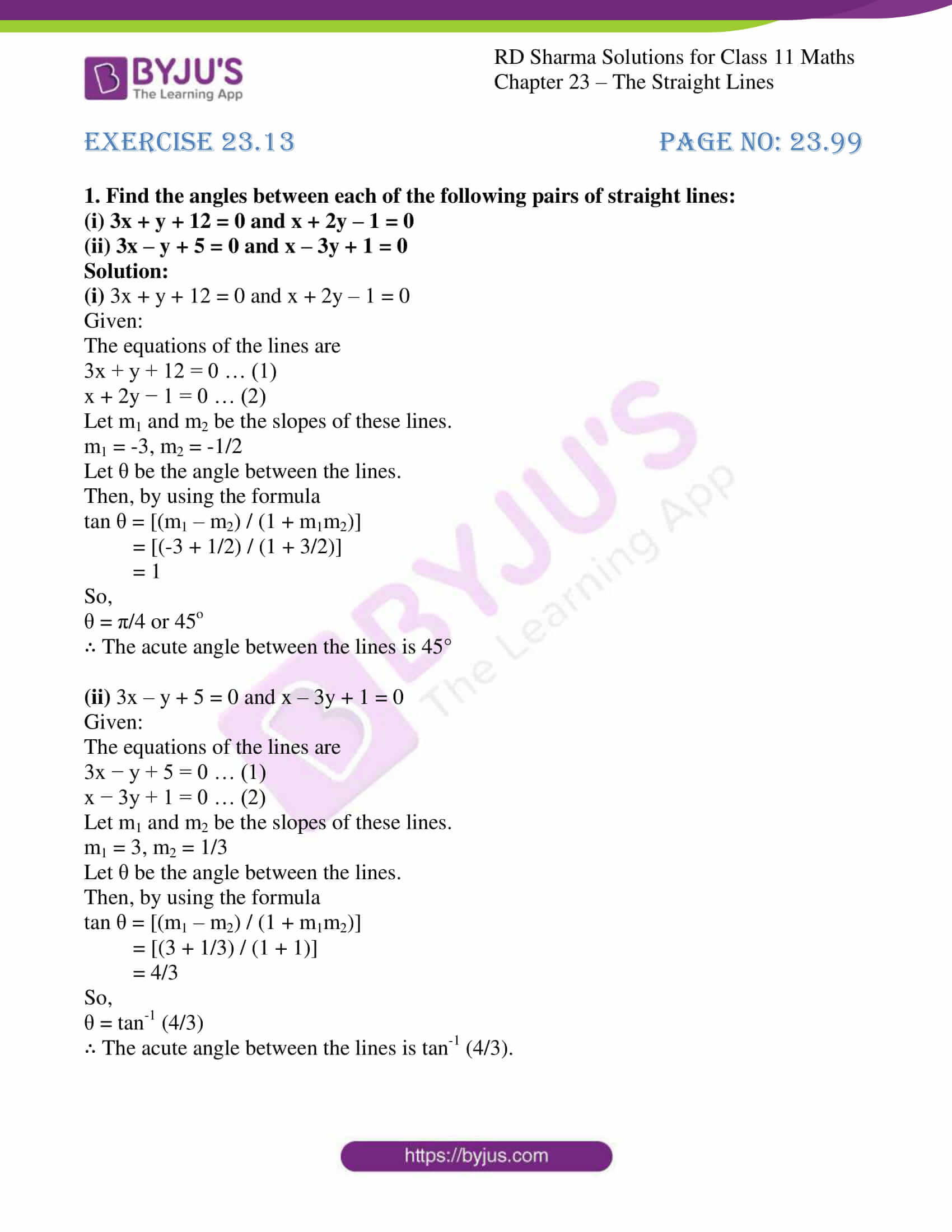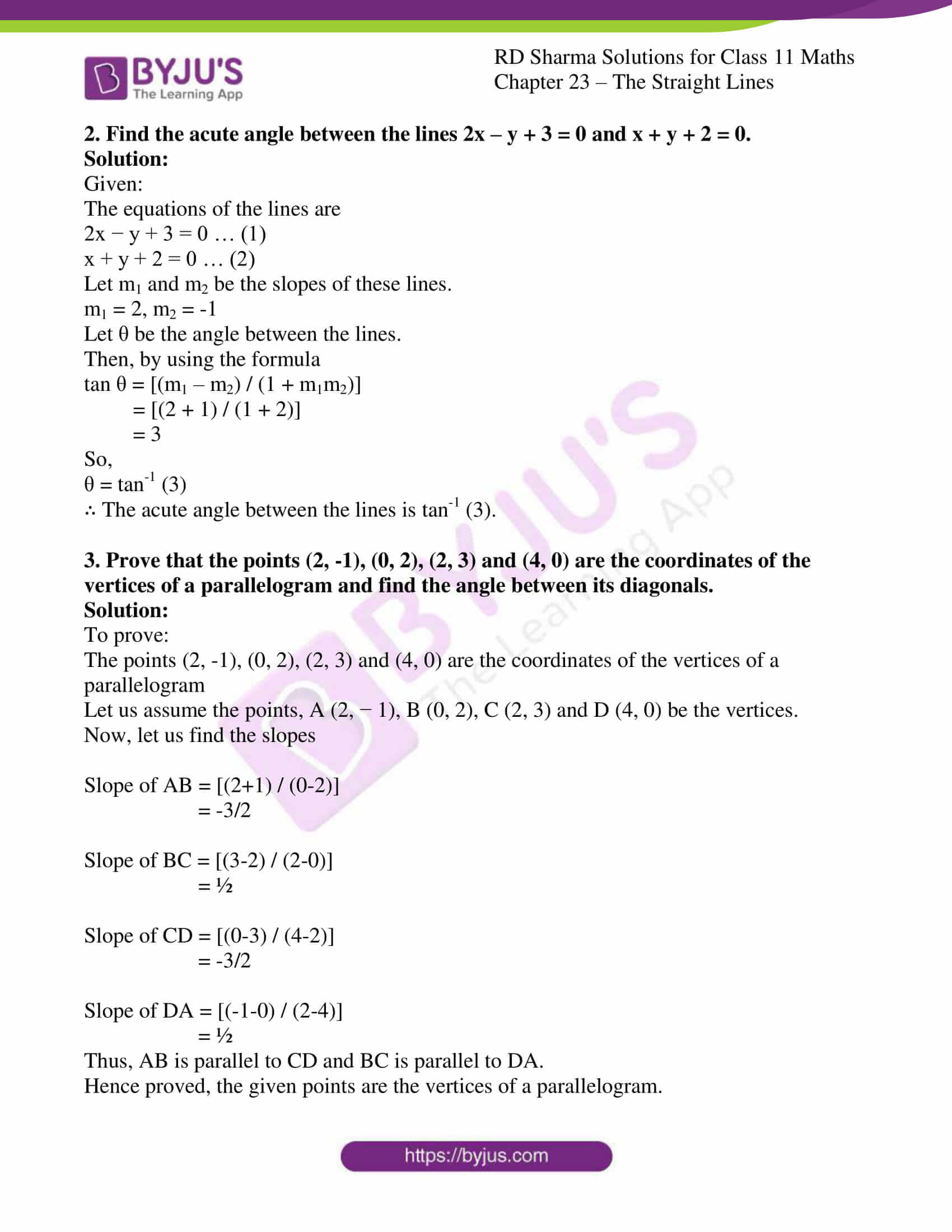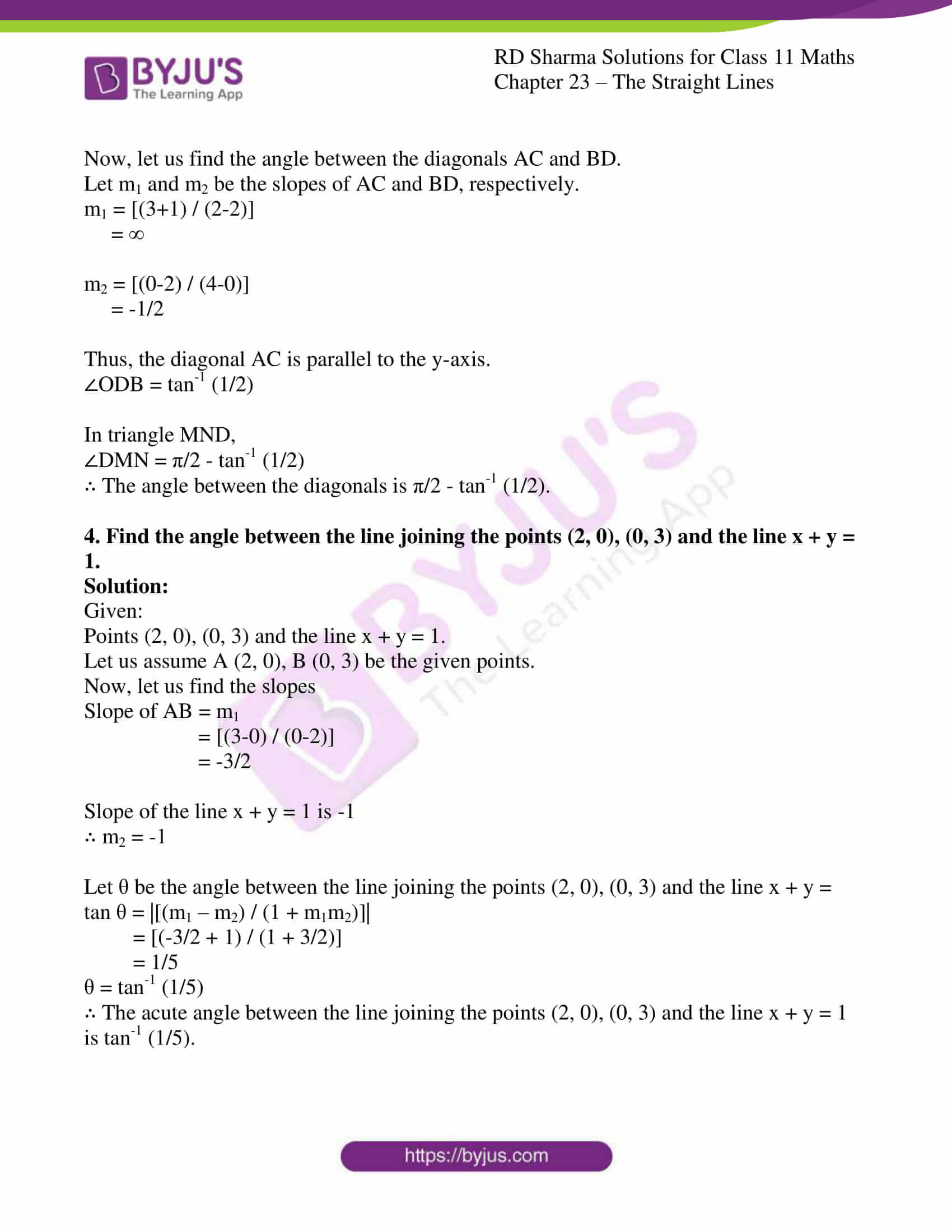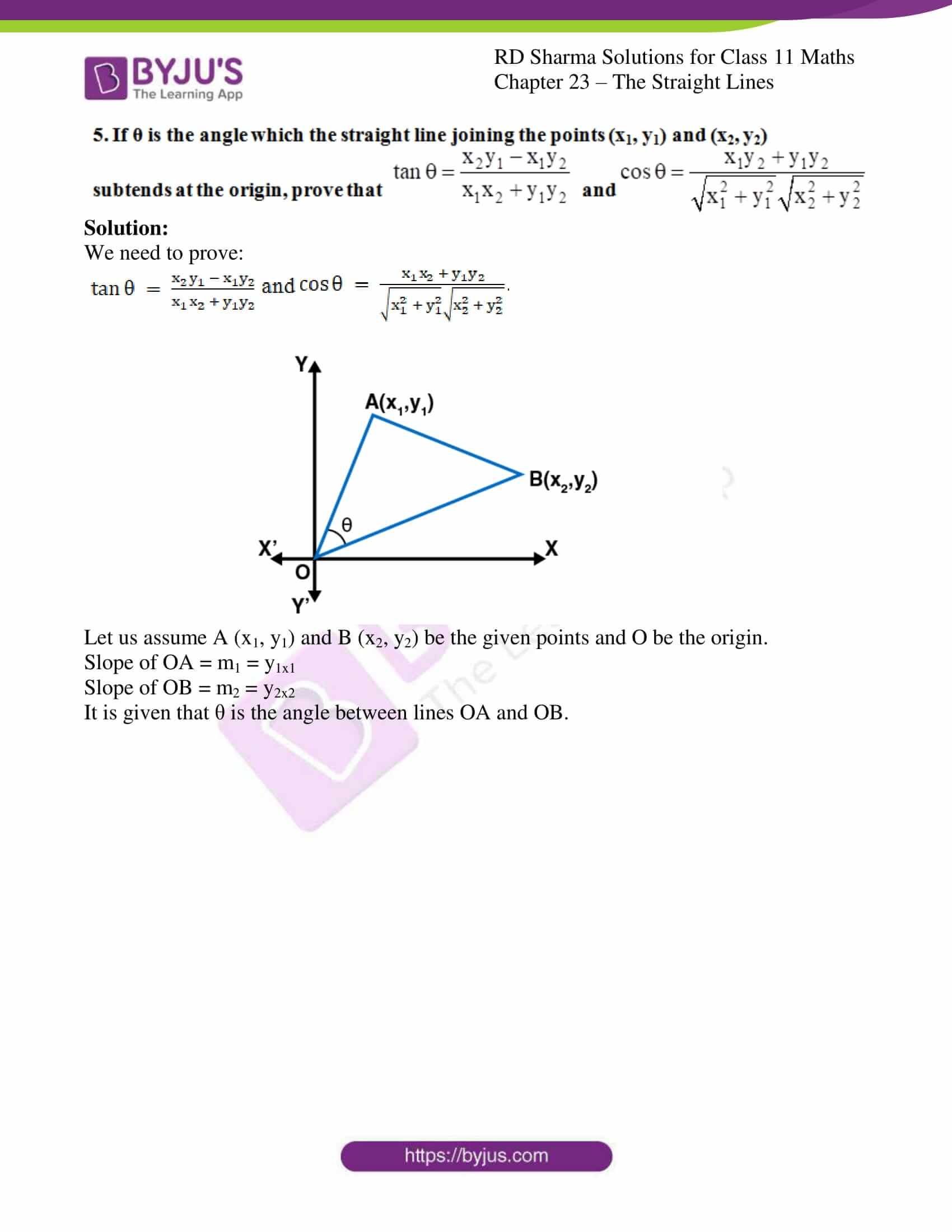### Also, access other exercises of RD Sharma Solutions for Class 11 Maths Chapter 23 – The Straight Lines

Exercise 23.1 Solutions

Exercise 23.2 Solutions

Exercise 23.3 Solutions

Exercise 23.4 Solutions

Exercise 23.5 Solutions

Exercise 23.6 Solutions

Exercise 23.7 Solutions

Exercise 23.8 Solutions

Exercise 23.9 Solutions

Exercise 23.10 Solutions

Exercise 23.11 Solutions

Exercise 23.12 Solutions

Exercise 23.14 Solutions

Exercise 23.15 Solutions

Exercise 23.16 Solutions

Exercise 23.17 Solutions

Exercise 23.18 Solutions

Exercise 23.19 Solutions

### Access answers to RD Sharma Solutions for Class 11 Maths Exercise 23.13 Chapter 23 – The Straight Lines

#### EXERCISE 23.13 PAGE NO: 23.99

1. Find the angles between each of the following pairs of straight lines:
(i) 3x + y + 12 = 0 and x + 2y – 1 = 0

(ii) 3x – y + 5 = 0 and x – 3y + 1 = 0

Solution:

(i) 3x + y + 12 = 0 and x + 2y – 1 = 0

Given:

The equations of the lines are

3x + y + 12 = 0 … (1)

x + 2y − 1 = 0 … (2)

Let m1 and m2 be the slopes of these lines.

m1 = -3, m2 = -1/2

Let θ be the angle between the lines.

Then, by using the formula

tan θ = [(m1 – m2) / (1 + m1m2)]

= [(-3 + 1/2) / (1 + 3/2)]

= 1

So,

θ = π/4 or 45o

∴ The acute angle between the lines is 45°

(ii) 3x – y + 5 = 0 and x – 3y + 1 = 0

Given:

The equations of the lines are

3x − y + 5 = 0 … (1)

x − 3y + 1 = 0 … (2)

Let m1 and m2 be the slopes of these lines.

m1 = 3, m2 = 1/3

Let θ be the angle between the lines.

Then, by using the formula

tan θ = [(m1 – m2) / (1 + m1m2)]

= [(3 + 1/3) / (1 + 1)]

= 4/3

So,

θ = tan-1 (4/3)

∴ The acute angle between the lines is tan-1 (4/3).

2. Find the acute angle between the lines 2x – y + 3 = 0 and x + y + 2 = 0.

Solution:

Given:

The equations of the lines are

2x − y + 3 = 0 … (1)

x + y + 2 = 0 … (2)

Let m1 and m2 be the slopes of these lines.

m1 = 2, m2 = -1

Let θ be the angle between the lines.

Then, by using the formula

tan θ = [(m1 – m2) / (1 + m1m2)]

= [(2 + 1) / (1 + 2)]

= 3

So,

θ = tan-1 (3)

∴ The acute angle between the lines is tan-1 (3).

3. Prove that the points (2, -1), (0, 2), (2, 3) and (4, 0) are the coordinates of the vertices of a parallelogram and find the angle between its diagonals.

Solution:

To prove:

The points (2, -1), (0, 2), (2, 3) and (4, 0) are the coordinates of the vertices of a parallelogram

Let us assume the points, A (2, − 1), B (0, 2), C (2, 3) and D (4, 0) be the vertices.

Now, let us find the slopes

Slope of AB = [(2+1) / (0-2)]

= -3/2

Slope of BC = [(3-2) / (2-0)]

= ½

Slope of CD = [(0-3) / (4-2)]

= -3/2

Slope of DA = [(-1-0) / (2-4)]

= ½

Thus, AB is parallel to CD and BC is parallel to DA.

Hence proved, the given points are the vertices of a parallelogram.

Now, let us find the angle between the diagonals AC and BD.

Let m1 and m2 be the slopes of AC and BD, respectively.

m1 = [(3+1) / (2-2)]

= ∞

m2 = [(0-2) / (4-0)]

= -1/2

Thus, the diagonal AC is parallel to the y-axis.

∠ODB = tan-1 (1/2)

In triangle MND,

∠DMN = π/2 – tan-1 (1/2)

∴ The angle between the diagonals is π/2 – tan-1 (1/2).

4. Find the angle between the line joining the points (2, 0), (0, 3) and the line x + y = 1.

Solution:

Given:

Points (2, 0), (0, 3) and the line x + y = 1.

Let us assume A (2, 0), B (0, 3) be the given points.

Now, let us find the slopes

Slope of AB = m1

= [(3-0) / (0-2)]

= -3/2

Slope of the line x + y = 1 is -1

∴ m2 = -1

Let θ be the angle between the line joining the points (2, 0), (0, 3) and the line x + y =

tan θ = |[(m1 – m2) / (1 + m1m2)]|

= [(-3/2 + 1) / (1 + 3/2)]

= 1/5

θ = tan-1 (1/5)

∴ The acute angle between the line joining the points (2, 0), (0, 3) and the line x + y = 1 is tan-1 (1/5).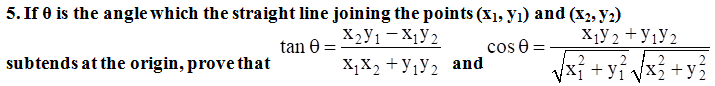Solution:

We need to prove: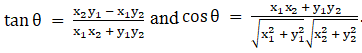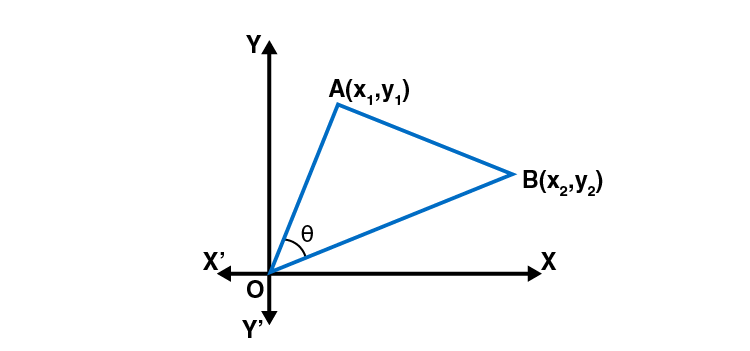Let us assume A (x1, y1) and B (x2, y2) be the given points and O be the origin.

Slope of OA = m1 = y1×1

Slope of OB = m2 = y2×2

It is given that θ is the angle between lines OA and OB.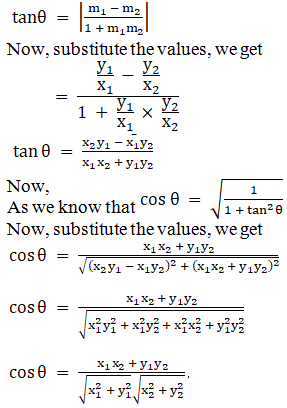Hence proved.